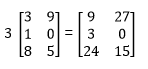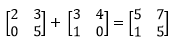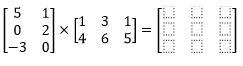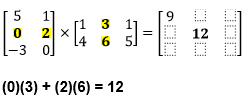Start Magoosh SAT or Magoosh ACT Prep today!Lots of students have minor panic attacks when they are first faced with matrices on the ACT Math Test, but never fear! Even if matrices look completely foreign to you, a quick read through this blog will calm your nerves. 🙂

Luckily, matrix questions are quite rare on the ACT (you might not see a single one throughout the 60 questions on the ACT Math Test!), and require only basic addition and subtraction skills (or *cough* a TI-83 calculator *cough*) to solve. If you can add 5 and 6, you’ll be fine!

If you just need a quick refresher, check out the video below. Otherwise, read on!

## ACT Matrix Basics

The word matrix refers to a rectangular-looking box filled with numbers arranged in rows and columns Each number in the matrix is called an element. When it comes to matrices on the test, you really just need to know the basics:

## First of All, Matrices Are Referred to by Their Dimensions.

Matrices can come in all sorts of sizes. We write the size of a matrix first with its number of rows and then its number of columns.

This, for example, is a 3 x 2 matrix. 3 rows and 2 columns:## Scalar Multiplication

You might see a question on the ACT that asks you do what is called scalar multiplication. This means that we are multiplying a matrix by an ordinary number. Every entry inside the matrix just gets multiplied by that number.You might also see a question on the ACT on adding or subtracting matrices. Important note: you can only add or subtract two matrices of exactly the same dimensions. We add or subtract matrices by adding or subtracting the corresponding numbers (the numbers that are in the same “spot” on each matrix).Notice that the size of the matrices is the same, and that each element in the first matrix is added to the corresponding element in the second matrix to get the corresponding element in the third matrix. -3 + -2 = -5.

With that understanding, we can solve for x by writing a simple equation: x + 9 = 11; x = 2.

## Multiplying Matrices

All right, I saved the trickiest for last. You can only multiply matrices if the number of columns in first matrix equals the number of rows in the second matrix. When we multiply matrices, the product matrix will have the same number of rows as first matrix and the same number of columns as the second. For example, the product of a 2 x 3 matrix and a 3 x 2 matrix would be a 3 x 3 matrix.In order to find the entry in the first column, first row of the product matrix, we are going to multiply each number in the first row of the first matrix by its corresponding number in the first column of the second matrix and add the products together.For the entry in the second row, second column, we will do the same thing with the second row of the first matrix and the second column of the second matrix:In order to completely fill out this matrix, we would have to do 9 calculations, but don’t fret, the ACT is more likely to give you smaller matrices or matrices with more zeros or ask you to find just one entry in a matrix.

Let’s look at a question involving subtracting matrices.

## Guided ACT Matrix Practice QuestionWhat is the sum of x and y?

To answer this, we will set up two equations to solve for x and y independently.

16 – 2 = x and 3 – (-4) = y

14 = x and 7 = y

Their sum is 14 + 7 = 21.

Sometimes a variable will be introduced to stand for an entire matrix. Let’s look at an example:Carefully subtract each corresponding element.Now try a practice question on your own!

## More ACT Matrix Practice QuestionsWhat is the solution to the matrices?To subtract matrices, subtract each corresponding element, as shown above. Remember that subtracting a negative number becomes addition.

With practice, you’ll see that the math involved in ACT matrix questions is rarely challenging – just keep the rows and columns lined up and you can’t help but get them right!

#### Improve your SAT or ACT score, guaranteed. Start your 1 Week Free Trial of Magoosh SAT Prep or your 1 Week Free Trial of Magoosh ACT Prep today!Mike creates expert lessons and practice questions to guide GMAT students to success. He has a BS in Physics and an MA in Religion, both from Harvard, and over 20 years of teaching experience specializing in math, science, and standardized exams. Mike likes smashing foosballs into orbit, and despite having no obvious cranial deficiency, he insists on rooting for the NY Mets.

### 2 Responses to “Matrices on the ACT”

1.Mekha Thomas says:

The first matrix example in the video was a 2×3 matrix not a 3X2 matrix, I think the numbers were switched around, Otherwise the video was wonderful!

•Magoosh Test Prep Expert says:

Hi Mekha!

Thanks for pointing this out. 🙂 I’ll make a note of this for our content team so they can look into it. With that said, I’m glad you enjoyed the video!

Magoosh blog comment policy: To create the best experience for our readers, we will approve and respond to comments that are relevant to the article, general enough to be helpful to other students, concise, and well-written! :) If your comment was not approved, it likely did not adhere to these guidelines. If you are a Premium Magoosh student and would like more personalized service, you can use the Help tab on the Magoosh dashboard. Thanks!